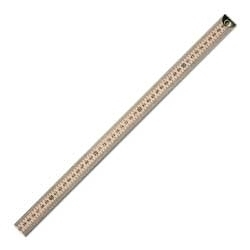# How Big is a Meter

Every meter stick reveals a meter scale, that’s why it is called so. How big is a meter? How important is this unit of measurement? Well, we give you answers right here.

### How big is a Meter

A meter is a unit of measurement in the Metric system. There are 39.37 inches, a hundred centimeters, and a little over three feet to a meter. Yes, it is not that big. Remember that there is still the kilometer, miles, etc. But it is definitely bigger than a centimeter, an inch, a foot, and even a yard.

A meter is so important when you are measuring in Metric system and you are serious about getting accurate results. You need to get used to this measurement system and practice using it day-by-day to get the hang of it.

To read a measurement in meters, the first thing to do is to flip the meter stick where the meter scale is printed. Usually, on the other side of it is the centimeter scale with smaller lines in between for millimeters. The meter scale also has lines in between for measuring centimeters.

Next up, lay down the object you wish to measure. Line up the meter stick from the start point at the 0 scale, then mark the end point.

Count the meter lines from the start point to the end point of the object you are measuring. The number of those lines reveals the measurement in meters. If it is necessary, count the centimeter and millimeter millimeter lines as well. Then, convert your taken measurement into decimals or into centimeters, if that is your preferred unit.### When a Meter Matters

A meter is about 3.2808399 feet. When are you going to use this unit of measurement? When does a meter matter?

Well, measuring in meters is significant in more ways than one. It is quite necessary when building a house, when buying a new furniture piece, when running on tracks, when swimming on the pool, when driving a car. It is actually helpful to know how to measure in meters for everyday use. A lot of things would require you to take the right measurements. A lot of things that you do in your life require that you know how to measure. It is also important that you don’t only know the conversion system by heart but also understand it.

Every unit of measurement matters because they all make up an accurate measurement. Without one or the other, it could be difficult to tell an accurate measurement when it is important.